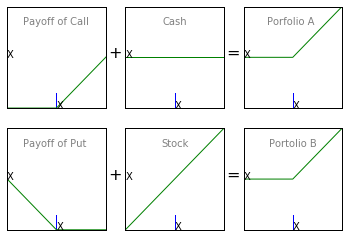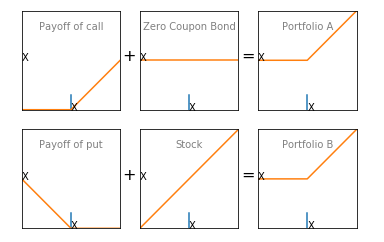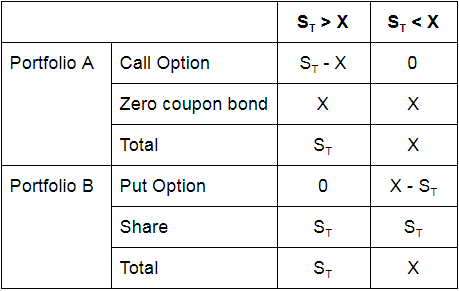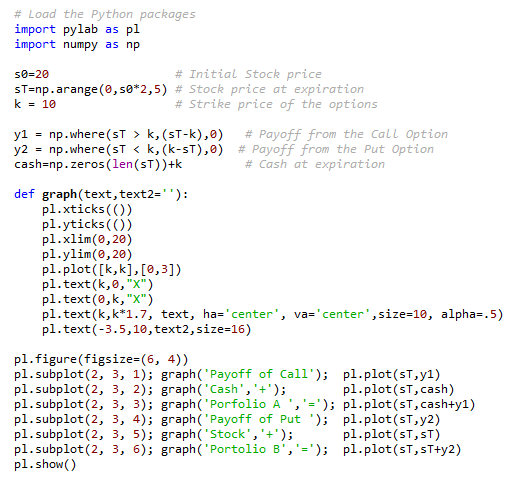# Put-Call Parity In PythonPut-call parity is a concept that anyone who is interested in options trading needs to understand. By gaining an understanding of put-call parity you can understand how the value of call option, put option and the stock are related to each other. This enables you to create other synthetic position using various option and stock combination. So let’s start this article by defining the put-call parity principle.

Put-call parity principle defines the relationship between the price of a European Put option and European Call option, both having the same underlying asset, strike price and expiration date.

If there is a deviation from put-call parity, then it would result in an arbitrage opportunity. Traders would take advantage of this opportunity to make riskless profits till the time the put-call parity is established again.

The put-call parity principle can be used to validate an option pricing model. If the option prices as computed by the model violate the put-call parity rule, such a model can be considered to be incorrect.

### Understanding Put-Call Parity

To understand put-call parity, consider a portfolio “A” comprising of a call option and cash. The amount of cash held equals the call strike price. Consider another portfolio “B” comprising of a put option and the underlying asset. S0 is the initial price of the underlying asset and ST is its price at expiration. Let “r” be the risk-free rate and “T” be the time for expiration. In time “T” the Zero coupon bond will be worth K (strike price) given the risk-free rate of “r”.

Portfolio A = Call option + Zero coupon bond
Portfolio B = Put option + Underlying AssetIf the share price is higher than X the call option will be exercised. Else, cash will be retained. Hence, at “T” portfolio A’s worth will be given by max(ST, X).

If the share price is lower than X, the put option will be exercised. Else, the underlying asset will be retained. Hence, at “T”, portfolio B’s worth will be given by max (ST, X).

If the two portfolios are equal at time “T”, then they should be equal at any time. This gives us the put-call parity equation :

C + Xe-rT = P + S0

In this equation,

• C is the premium on European Call Option
• P is the premium of European Put Option
• Sis the spot price of underlying stock
• And, Xe-rT is the current value (discounted value) of Zero coupon bond (X)
We can summarize the payoffs of both the portfolios under different conditions as shown in the table below.From the above table, we can see that under both the scenarios, the payoffs from both the portfolios are equal.

### Required Conditions For Put-call Parity

For put-call parity to hold, following conditions should be met. However, in the real world, they hardly hold true and put-call parity equation may need some modifications accordingly. For the purpose of this blog, we have assumed that these conditions are met.
• The underlying stock doesn’t pay any dividend during the life of the European options
• There are no transaction costs
• There are no taxes
• Shorting is allowed and there are no borrow charges
Hence, put-call parity will hold in a frictionless market with the underlying stock paying no dividends.

### Arbitrage Opportunity

When put-call parity principle gets violated, traders will try to take advantage of the arbitrage opportunity. An arbitrage trader will go long on the undervalued portfolio and short the overvalued portfolio to make a risk-free profit.

Let us now consider an example with some numbers to see how a trade can take advantage of arbitrage opportunities.

Let’s assume that the spot price of a stock is \$31, the risk-free interest rate is 10% per annum, the premium on three-month European call and put are \$3 and \$2.25 respectively and the exercise price is \$30.

In this case, the value of portfolio A will be,

C+Xe-rT = 3+30e-0.1 * 3/12 = \$32.26

The value of the portfolio B will be,

P+S0=2.25+31 = \$33.25

Portfolio B is overvalued and hence an arbitrageur can earn by going long on portfolio A and short on portfolio B. The following steps can be followed to earn the arbitrage profits.
1. Short the stock. This will generate a cash inflow of \$31.
2. Short the put option. This will generate a cash inflow of \$2.25.
3. Purchase the call option. This will generate cash outflow of \$3.
4. Total cash inflow is -3 + 2.25 + 31 = \$30.25. Invest \$30.25 in a zero coupon bond with 3 months maturity with a yield of 10% per annum.
Return from the zero coupon bond after three months will be 30.25e 0.1 * 3/12 = \$31.02.

If the stock price at maturity is above \$30, the call option will be exercised and if the stock price is less than \$30, the put option will be exercised. In both the scenarios, the arbitrageur will buy one stock at \$30. This stock will be used to cover the short.

Total profit from the arbitrage = \$31.02 - \$30 = \$1.02

### Python Codes Used For Plotting The Charts:

The below code can be used to plot the payoffs of the portfolios.### Summary

To summarize, we have seen the basic put-call parity equation. We have graphically as well as analytically seen how the equation holds true in real world. We have also covered the python codes to plot payoffs. We have then discussed the assumptions and moved on to see how a trader can look for arbitrage opportunities if market imperfections exist.

### Next Step

If you want to learn various aspects of Algorithmic trading then check out the Executive Programme in Algorithmic Trading (EPAT™). The course covers training modules like Statistics & Econometrics, Financial Computing & Technology, and Algorithmic & Quantitative Trading. EPAT™ equips you with the required skill sets to be a successful trader. Enroll now!

Disclaimer: All investments and trading in the stock market involve risk. Any decisions to place trades in the financial markets, including trading in stock or options or other financial instruments is a personal decision that should only be made after thorough research, including a personal risk and financial assessment and the engagement of professional assistance to the extent you believe necessary. The trading strategies or related information mentioned in this article is for informational purposes only.